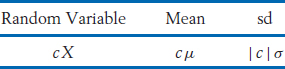# Expectation and Standard Deviation—Properties

The expected value of a discrete random variable is a summation of the products (value × probability). The key properties of expectations are then all inherited from the properties of summation. In this appendix, we indicate this development for some of the most useful properties of expectation and variance. The interested reader can consult Bhattacharyya and Johnson1 for more details.

A3.1 EXPECTED VALUE AND STANDARD DEVIATION OF cX + b

The units of the random variable X may be changed by multiplying by a constant, for example,or by adding a constant, for example,The mean and standard deviation of the new random variables are related to μ = E (X) and σ = sd (X).

If X is multiplied by a constant c,If a constant b is added to X,Notice that adding a constant to a random variable leaves the standard deviation unchanged.

Example

Let X have mean = 3 = μ and standard deviation = 5 = σ. Find the mean and sd of (a) X + 4, (b) 2X, (c) −X, and (d) .

SOLUTION

By the foregoing properties,

Finally, (X − 3) has mean 3 − 3 = 0 and sd ...

Get Statistics: Principles and Methods, 7th Edition now with the O’Reilly learning platform.

O’Reilly members experience books, live events, courses curated by job role, and more from O’Reilly and nearly 200 top publishers.